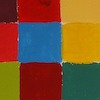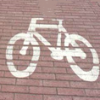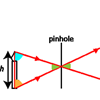Resources tagged with: Similarity and congruence

Filter by: Content type:
Age range:
Challenge level:

There are 45 results

Broad Topics > Angles, Polygons, and Geometrical Proof > Similarity and congruenceFrom All Corners

Age 14 to 16Challenge Level

Straight lines are drawn from each corner of a square to the mid points of the opposite sides. Express the area of the octagon that is formed at the centre as a fraction of the area of the square.Figure of Eight

Age 14 to 16Challenge Level

On a nine-point pegboard a band is stretched over 4 pegs in a "figure of 8" arrangement. How many different "figure of 8" arrangements can be made ?Wedge on Wedge

Age 14 to 16Challenge Level

Two right-angled triangles are connected together as part of a structure. An object is dropped from the top of the green triangle where does it pass the base of the blue triangle?Trapezium Four

Age 14 to 16Challenge Level

The diagonals of a trapezium divide it into four parts. Can you create a trapezium where three of those parts are equal in area?Arrh!

Age 14 to 16Challenge Level

Triangle ABC is equilateral. D, the midpoint of BC, is the centre of the semi-circle whose radius is R which touches AB and AC, as well as a smaller circle with radius r which also touches AB and AC. . . .Triangular Slope

Age 14 to 16 ShortChallenge Level

Can you find the gradients of the lines that form a triangle?Triangle in a Triangle

Age 14 to 16Challenge Level

Can you work out the fraction of the original triangle that is covered by the inner triangle?Nicely Similar

Age 14 to 16Challenge Level

If the hypotenuse (base) length is 100cm and if an extra line splits the base into 36cm and 64cm parts, what were the side lengths for the original right-angled triangle?Napkin

Age 14 to 16Challenge Level

A napkin is folded so that a corner coincides with the midpoint of an opposite edge . Investigate the three triangles formed .Age 14 to 16Challenge Level

Two ladders are propped up against facing walls. The end of the first ladder is 10 metres above the foot of the first wall. The end of the second ladder is 5 metres above the foot of the second. . . .Triangular Tantaliser

Age 11 to 14Challenge Level

Draw all the possible distinct triangles on a 4 x 4 dotty grid. Convince me that you have all possible triangles.Triangle in a Trapezium

Age 11 to 16Challenge Level

Can you find and prove the relationship between the area of a trapezium and the area of a triangle constructed within it?Age 14 to 16Challenge Level

Find the area of the shaded region created by the two overlapping triangles in terms of a and b?Doubly Isosceles

Age 14 to 16 ShortChallenge Level

Find the missing distance in this diagram with two isosceles trianglesLook Before You Leap

Age 14 to 16Challenge Level

Can you spot a cunning way to work out the missing length?Triangle Midpoints

Age 14 to 16Challenge Level

You are only given the three midpoints of the sides of a triangle. How can you construct the original triangle?The Square Under the Hypotenuse

Age 14 to 16Challenge Level

Can you work out the side length of a square that just touches the hypotenuse of a right angled triangle?Sitting Pretty

Age 14 to 16Challenge Level

A circle of radius r touches two sides of a right angled triangle, sides x and y, and has its centre on the hypotenuse. Can you prove the formula linking x, y and r?A Sameness Surely

Age 14 to 16Challenge Level

Triangle ABC has a right angle at C. ACRS and CBPQ are squares. ST and PU are perpendicular to AB produced. Show that ST + PU = ABPoints in Pairs

Age 14 to 16Challenge Level

Move the point P to see how P' moves. Then use your insights to calculate a missing length.It Depends on Your Point of View!

Age 14 to 16Challenge Level

Anamorphic art is used to create intriguing illusions - can you work out how it is done?Making Sixty

Age 14 to 16Challenge Level

Why does this fold create an angle of sixty degrees?Pythagoras Proofs

Age 14 to 16Challenge Level

Can you make sense of these three proofs of Pythagoras' Theorem?Folding Fractions

Age 14 to 16Challenge Level

What fractions can you divide the diagonal of a square into by simple folding?Folding Squares

Age 14 to 16Challenge Level

The diagonal of a square intersects the line joining one of the unused corners to the midpoint of the opposite side. What do you notice about the line segments produced?How Big?

Age 11 to 14Challenge Level

If the sides of the triangle in the diagram are 3, 4 and 5, what is the area of the shaded square?Partly Circles

Age 14 to 16Challenge Level

What is the same and what is different about these circle questions? What connections can you make?Slippage

Age 14 to 16Challenge Level

A ladder 3m long rests against a wall with one end a short distance from its base. Between the wall and the base of a ladder is a garden storage box 1m tall and 1m high. What is the maximum distance. . . .Kite in a Square

Age 14 to 16Challenge Level

Can you make sense of the three methods to work out the area of the kite in the square?Squirty

Age 14 to 16Challenge Level

Using a ruler, pencil and compasses only, it is possible to construct a square inside any triangle so that all four vertices touch the sides of the triangle.Fit for Photocopying

Age 14 to 16Challenge Level

Explore the relationships between different paper sizes.Parallel Universe

Age 14 to 16Challenge Level

An equilateral triangle is constructed on BC. A line QD is drawn, where Q is the midpoint of AC. Prove that AB // QD.Fitting In

Age 14 to 16Challenge Level

The largest square which fits into a circle is ABCD and EFGH is a square with G and H on the line CD and E and F on the circumference of the circle. Show that AB = 5EF. Similarly the largest. . . .Same Length

Age 11 to 16Challenge Level

Construct two equilateral triangles on a straight line. There are two lengths that look the same - can you prove it?Overlap

Age 11 to 14Challenge Level

A red square and a blue square overlap so that the corner of the red square rests on the centre of the blue square. Show that, whatever the orientation of the red square, it covers a quarter of the. . . .Two Triangles in a Square

Age 14 to 16Challenge Level

Given that ABCD is a square, M is the mid point of AD and CP is perpendicular to MB with P on MB, prove DP = DC.Angle Trisection

Age 14 to 16Challenge Level

It is impossible to trisect an angle using only ruler and compasses but it can be done using a carpenter's square.Matter of Scale

Age 14 to 16Challenge Level

Prove Pythagoras' Theorem using enlargements and scale factors.Hex

Age 11 to 14Challenge Level

Explain how the thirteen pieces making up the regular hexagon shown in the diagram can be re-assembled to form three smaller regular hexagons congruent to each other.Pentakite

Age 14 to 18Challenge Level

ABCDE is a regular pentagon of side length one unit. BC produced meets ED produced at F. Show that triangle CDF is congruent to triangle EDB. Find the length of BE.Pent

Age 14 to 18Challenge Level

The diagram shows a regular pentagon with sides of unit length. Find all the angles in the diagram. Prove that the quadrilateral shown in red is a rhombus.Bus Stop

Age 14 to 16Challenge Level

Two buses leave at the same time from two towns Shipton and Veston on the same long road, travelling towards each other. At each mile along the road are milestones. The buses' speeds are constant. . . .All Tied Up

Age 14 to 16Challenge Level

A ribbon runs around a box so that it makes a complete loop with two parallel pieces of ribbon on the top. How long will the ribbon be?